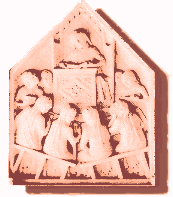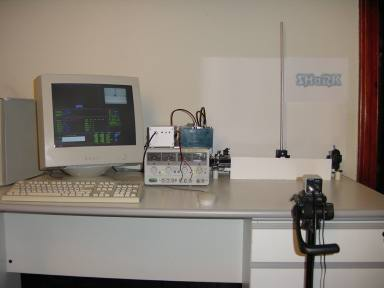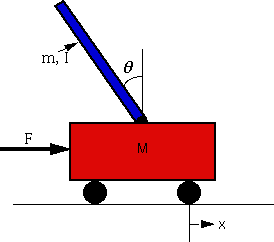#Real-Time  Inverted PendulumPresentation of the experiment

To see a presentation with the details of the apparatus and some experimental results, click here

Pendulum stabilization

## INTRODUCTION

Inverted Pendulum Experiment implemented at RetisLab in Pisa.

### What is an inverted pendulum?An inverted pendulum is a physical device consisting in a cylinrical  bar (usually of alluminum) free to oscillate around a fixed pivot. The pivot is mounted on a carriage, which in its turn can move on a horizontal direction.
The carriage is driven by a motor, which can exert on it a variable force.
The bar would naturally tend to fall down from the top vertical position, which is a position of  unsteady equilibrium.

The goal of the experiment is to stabilize the pendulum (bar) on the top vertical position.
This  is possible by exerting on the carriage through the motor a force which tends to contrast the 'free' pendulum dynamics.
The correct force has to be calculated measuring the instant values of the horizontal position and the pendulum angle (obtained e.g. through two potentiometers).

The system pendulum+cart+motor can be modeled as a linear system if all the parameters are known (masses, lengths, etc.), in order to find a controller to stabilize it. If not all the parameters are known, one can however try to 'reconstruct' the system parameters using measured data on the dynamics of the pendulum.

### What is it used for?

The inverted pendulum is a traditional example ( neither difficult nor trivial) of a controlled system.
Thus it is used in simulations and experiments to show the performance of different controllers ( e.g. PID controllers, state space controllers, fuzzy controllers....).

The Real-Time Inverted Pendulum is used as a benchmark, to test the validity and the performance of the software underlying the state-space controller alogorithm, i.e. the used operating system.
Actually the algorithm is implement form the numerical point of view as a set of mutually co-operating tasks, which are periodically activated by the kernel, and which perform different calculations.
The way how these tasks are activated (e.g. the activation order) is calleding scheduling of the tasks.
It is obvious that a correct scheduling of each task is crucial for a good performance of the controller, and hence for an effective pendulum stabilization.
Thus the inverted pendulum is very useful in determing whether a particular schedulig choice is better than another one, in which cases, to which extent, and so on.

To see a more detailed presentation of the system, and some experimental results, click here.

### Modeling an inverted pendulum

Generally the inverted pendulum system is modeled as a linear system, and hence the modeling is valid only for small oscillations of the pendulum.
A sort of 'tutorial' on the modeling of an inverted pendulum expoiting Matlab can be found here.
In the implementation of our control system we used a stete-space design; an explanation of a state space design for an inverted pendulum can be found here.
Further control tutorials -not on the inverted pendulum- using Matlab can be found  here.